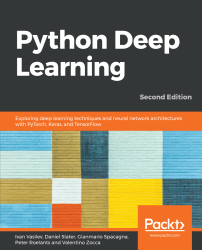•#### Python Deep Learning - Second Edition#### Overview of this book

With the surge in artificial intelligence in applications catering to both business and consumer needs, deep learning is more important than ever for meeting current and future market demands. With this book, you’ll explore deep learning, and learn how to put machine learning to use in your projects. This second edition of Python Deep Learning will get you up to speed with deep learning, deep neural networks, and how to train them with high-performance algorithms and popular Python frameworks. You’ll uncover different neural network architectures, such as convolutional networks, recurrent neural networks, long short-term memory (LSTM) networks, and capsule networks. You’ll also learn how to solve problems in the fields of computer vision, natural language processing (NLP), and speech recognition. You'll study generative model approaches such as variational autoencoders and Generative Adversarial Networks (GANs) to generate images. As you delve into newly evolved areas of reinforcement learning, you’ll gain an understanding of state-of-the-art algorithms that are the main components behind popular games Go, Atari, and Dota. By the end of the book, you will be well-versed with the theory of deep learning along with its real-world applications.
PrefaceFree Chapter
Machine Learning - an IntroductionNeural NetworksDeep Learning FundamentalsComputer Vision with Convolutional NetworksGenerating Images with GANs and VAEsRecurrent Neural Networks and Language ModelsReinforcement Learning TheoryDeep Reinforcement Learning for GamesDeep Learning in Autonomous VehiclesOther Books You May Enjoy# Finding optimal policies with Dynamic Programming

Dynamic Programming (DP) is a base for many RL algorithms. The main paradigm of DP algorithms is to use the state- and action-value functions as tools to find the optimal policy, given a fully-known model of the environment. In this section, we'll see how to do that.

# Policy evaluation

We'll start with policy evaluation, or how to compute the state-value function,, given a specific policy, π. This task is also known as prediction. As a reminder, we'll assume that the state-value function is a table. We'll implement policy evaluation using the state-value Bellman equation we defined in the Bellman equations section. Let's start:

1. Input the following...